### Home > CALC > Chapter 6 > Lesson 6.5.1 > Problem6-169

6-169.
1. Find the exact value of each of the following limits. Note that finding the exact value requires that the limit be found analytically rather than numerically. Homework Help ✎

1.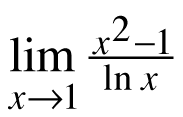2.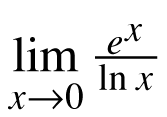3.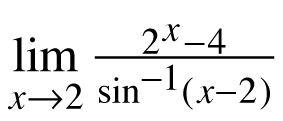4.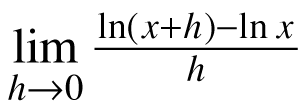5.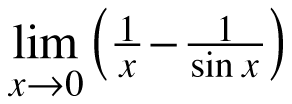6.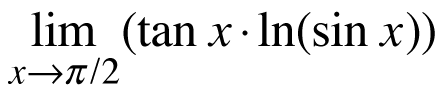7.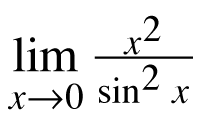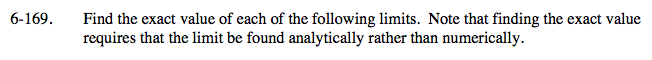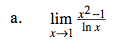$\rightarrow \frac{0}{0},\text{ use l'Hopital's Rule }$

$=\lim_{x\rightarrow 1}\frac{2x}{\frac{1}{x}}$

2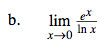Careful! Be sure to check:
For a limit to exist, the limit from the left must agree with the limit from the right.

$\text{We know that }\lim_{x\rightarrow 0^{+}}\frac{e^{x}}{\text{ln}x}\rightarrow \frac{1}{-\infty }=0,$

$\text{What does }\lim_{x\rightarrow 0^–}\frac{e^{x}}{\text{ln}x}=\underline{\ \ \ \ \ \ \ \ }?$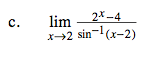$\rightarrow \frac{0}{0}$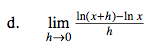Recognize that this is Hana's Definition of the Derivative, and find f '(x), or use l'Hopital's Rule.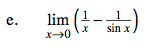→(0 – 0), which is also an indeterminate form.
Before using l'Hopital's Rule, you will need to convert the argument into a single fraction that

$\rightarrow \frac{0}{0} \text{ or }\frac{\infty }{\infty }.$

$=\lim_{x\rightarrow 0}\left ( \frac{1}{x}-\frac{1}{\text{sin}x} \right )=\lim_{x\rightarrow 0}\frac{\text{sin}x-x}{x\text{sin}x}\rightarrow \frac{0}{0}$

To solve this limit, you will have to use l'Hopital's Rule twice.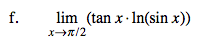$\text{To covert this limit into }\frac{0}{0}\text{ form, let tan}x=\frac{\text{sin}x}{\text{cos}x},$

then write the argument as a single fraction.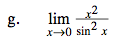See hint in part (a).